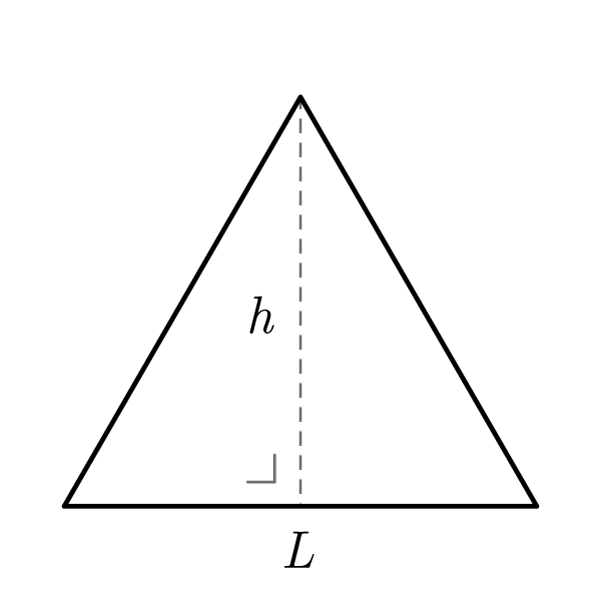# Equilateral Triangle

 Side $$S$$ Height $$h$$
$$2p = S \times 3$$
Perimeter
$$A = \frac{S \times h}{2}$$
Area
$$S = \frac{A \times 2}{h}$$
$$h = \frac{A \times 2}{S}$$
$$S = \frac{2p}{3}$$
Side
Knowing side or height only
$$2p = 2 h \sqrt{3}$$
Perimeter (with height)
$$h = \frac{2p}{2\sqrt{3}}$$
Height (from perimeter)
$$A = \frac{\sqrt{3}}{4} {S}^2$$
Area (with side)
$$A = \frac{{h}^2}{\sqrt{3}}$$
Area (with height)
$$S = \frac{2h}{\sqrt{3}}$$
Side (with height)
$$h = \frac{S\sqrt{3}}{2}$$
Height (with side)
Inscribed circumference
$$r = \frac{1}{2 \sqrt{3}} S$$
$$r = \frac{1}{3} h$$
$$S = 2r\sqrt{3}$$
Side
$$h = 3r$$
Height
$$2p = 6r\sqrt{3}$$
Perimeter
$$A = 3{r}^2 \sqrt{3}$$
Area
Circumscribed circumference
$$R = \frac{\sqrt{3}}{3} S$$
$$R = \frac{2}{3} h$$
$$S = R\sqrt{3}$$
Side
$$h = \frac{3}{2}R$$
Height
$$2p = 3\sqrt{3}R$$
Perimeter
$$A = \frac{3\sqrt{3}}{4}{R}^2$$
Area

## Definition

An equilateral triangle is a triangle with all sides congruent.

## Properties

1. All sides are congruent
2. All angles are congruent
3. All the  Generic Triangle formulas are valid
4. The height relative to the base divides the shape in two congruent right triangles. For these are valid the  Right Triangle formulasEquilateral Triangle Formulas
Data Formula
Perimeter 2p = S × 3
Area A = (S × h) / 2
Side S = 2p / 3
Side (with height) S = (2 × h) / √3
Height (with side) h = (S√3) / 2
Height (from perimeter) h = 2p / (2√3)
Perimeter (with height) A = 2 × h × √3
Area (with side) A = (√3 / 4) × S2
Area (with height) A = h2 / √3
Side S = (A × 2) / h
Height h = (A × 2) / S
Equilateral Triangle - Inscribed circumference
Data Formula
Inscribed radius (with side) r = [1 / (2√3)] × S
Inscribed radius (with height) r = 1/3 × h
Side S = 2r√3
Height h = 3r
Perimeter 2p = 6r√3
Area A = 3r2√3
Equilateral Triangle - Circumscribed circumference
Data Formula
Circumscribed radius (with side) r = (√3 / 3) × S
Circumscribed radius (with height) r = 2/3 × h
Side S = R√3
Height h = 3/2 × R
Perimeter 2p = 3√3R
Area A = [(3√3) / 4] × R2